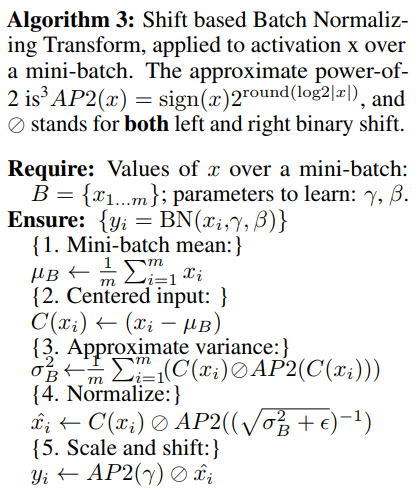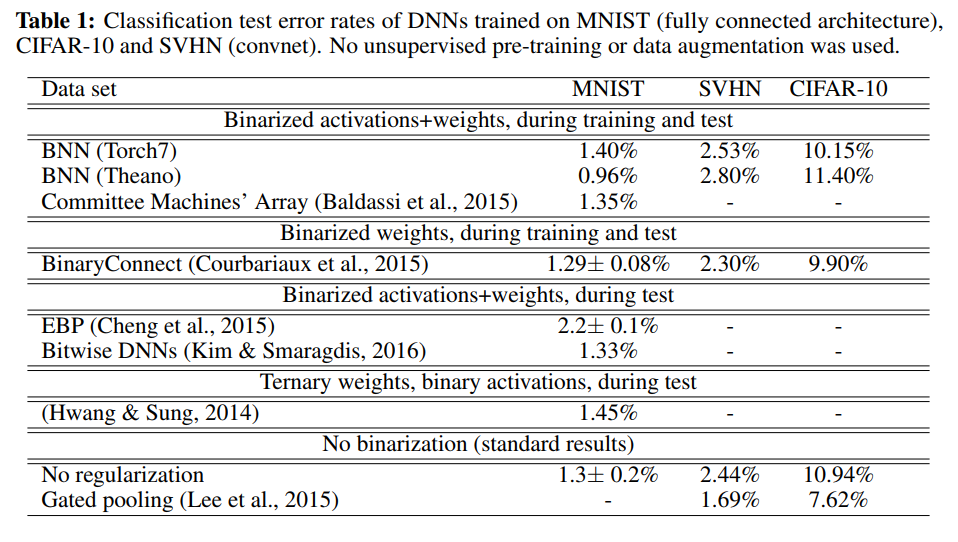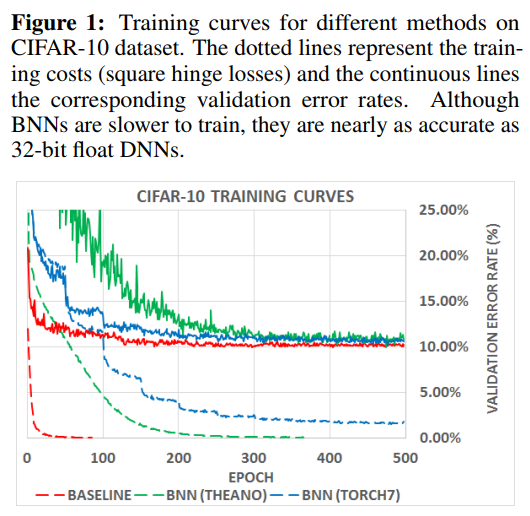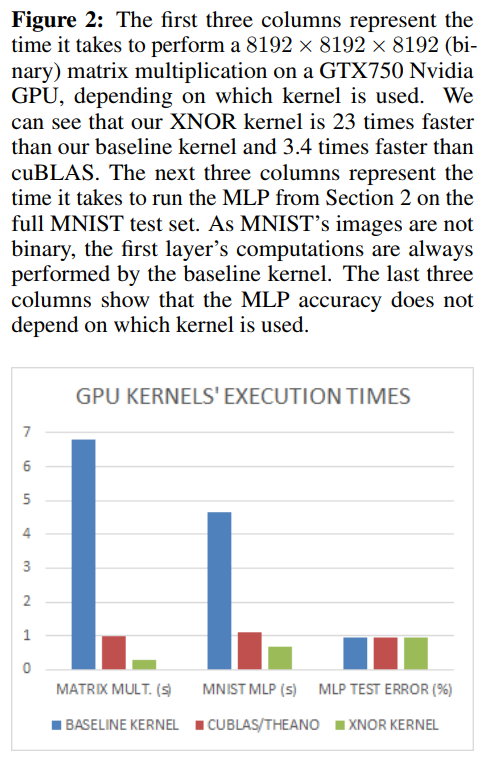## Summary

BNNs achieved nearly state-of-the-art results over the MNIST, CIFAR-10 and SVHN datasets We wrote a binary matrix multiplication GPU kernel with which it is possible to run our MNIST BNN 7 times faster than with an unoptimized GPU kernel, without suffering any loss in classification accuracy.

Goal :

• Binarize weights and activations
• Be more efficient:

most of the 32-bit floating point multiply-accumulations are replaced by 1-bit XNOR-count operations

• Use less memory:

In comparison with 32-bit DNNs, BNNs require 32 times smaller memory size and 32 times fewer memory accesses

### Deterministic vs. stochastic binarization

Deterministic :

$x^b = \text{sign}(x) = \begin{cases} +1 \text{ if } x >= 0, \\ -1 \text{ otherwise} \end{cases}$

Stochastic :

$x^b = \begin{cases} +1 \text{ with probability } p = \sigma(x), \\ -1 \text{ with probability } 1 - p \end{cases};$ $\text{ where } \sigma(x) = \text{clip}(\frac{x+1}{2}, 0, 1) = \max(0, \min(1, \frac{x+1}{2}))$
• Note: Stochastic binarization should be better than deterministic binarization, but is harder to implement since it requires random bits.

• Real-valued gradients of the weights and activations are stored during training for SGD to work.

• The derivative of the sign function is zero almost everywhere, so the gradient cannot be used as is. Instead, a straight-through estimator is used, which corresponds to computing the gradient of the hard tanh: $$\text{Htanh}(x) = \text{clip}(x, -1, 1)$$

• For better efficiency, Shift-based BatchNorm is used, which is an approximation of BatchNorm that uses almost no multiplications.## Experiments

• MNIST
• CIFAR-10
• SVHNBNNs take longer to train, but are nearly as accurate:New binary kernel is 7x faster on GPU: